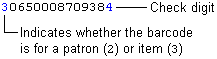## Ordering Barcodes and Patron Cards

You never want to find yourself without a good stash of barcodes for your items or barcoded patron cards.  These are custom orders that must be ordered in advance using particular prefix and formats to work in our Integrated Library System.  Your new barcodes will need to use your current prefix, and duplicate any existing records or unused supply you may yet use.

What do you need to know before you order?

1. What type of barcode do we use?
Codabar14 digit barcode, where the last digit is a check digit in a modulus 10 (Mod-10) complementary check digit format (more detail on this format).  When you are looking at the barcodes on a sheet, they may not look consecutive to you until you ignore that last number.
2. What is a check digit?
A check digit is the last digit in our codabar barcode.  It is the correct answer to an equation that is done on the previous 13 digits in the code.   It’s purpose is to check the validity of the barcode as it is scanned.  An incorrect answer would result in an error message, alerting you to rescan.
3. What your next number should be?
You wont want to repeat any number you have already used.  Create a list of all of your current records.  Sort the list by the barcode number.  Look at the first and last barcode number in your list.  The largest of the two numbers is the highest number that has been issued.  You will need to also check your current stock to find a higher number.  What is the next highest group that you would order.
For example if your highest barcode is 22390000027896, you might consider moving to the next decimal place and order 2239000010000#, where the last digit is the check digit.  In this example we just added started the next range by replacing the last 0 with a 1.

Document the order for the next time you need to order.

Barcode Validity Checking: An Example
A typical barcode consists of 14 digits in the following pattern:In this example, the barcode uses a modulus 10 (Mod-10) complementary check digit. The check digit derives from the barcode as follows:
Mod-10 Algorithms Differ Among Vendors

The method for calculating a Mod-10 check digit can differ from vendor to vendor. Check with your barcode vendor to determine if their algorithm matches the algorithm used here.

1. Starting with the second digit from the left, add every other digit. Using the example barcode above (without the check digit):2. Multiply each of the remaining digits by 2. If any product has two digits, add the digits to produce a single-digit number. Sum the results:3. Add the results from steps 1 and 2. In the example:
16 + 30 = 46
4. Subtract the units digit obtained in step 3 from the number 10. The result is the check digit. In the example:
10 – 6 = 4
4 is the check digit for the example barcode.